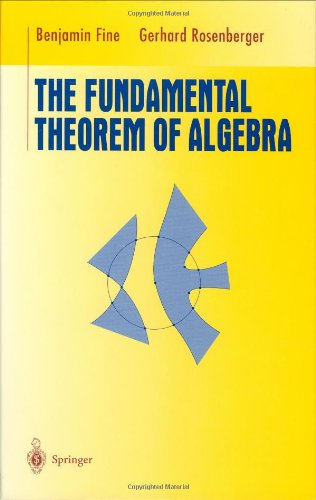# The Fundamental Theorem of Algebra (Undergraduate Texts in Mathematics)

## by Benjamin Fine

 Hardcover: 210 pages Publisher: Springer (Jun 20, 1997) Language: English ISBN-10: 0387946578 ISBN-13: 9780387946573
The fundamental theorem of algebra states that any complex polynomial must have a complex root. This book examines three pairs of proofs of the theorem from three different areas of mathematics: abstract algebra, complex analysis and topology. The first proof in each pair is fairly straightforward and depends only on what could be considered elementary mathematics. However, each of these first proofs leads to more general results from which the fundamental theorem can be deduced as a direct consequence. These general results constitute the second proof in each pair. To arrive at each of the proofs, enough of the general theory of each relevant area is developed to understand the proof. In addition to the proofs and techniques themselves, many applications such as the insolvability of the quintic and the transcendence of e and pi are presented. Finally, a series of appendices give six additional proofs including a version of Gauss'original first proof. The book is intended for junior/senior level undergraduate mathematics students or first year graduate students, and would make an ideal "capstone" course in mathematics.. ....
« Less More »Lowest New Price:  \$31.50
Lowest Used Price: \$33.97
View ListingWhile we make every effort to maintain the web site to ensure the accuracy of listed textbooks and prices, textbook requirements and prices are subject to change without notice.